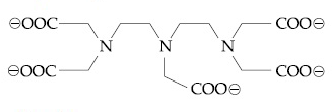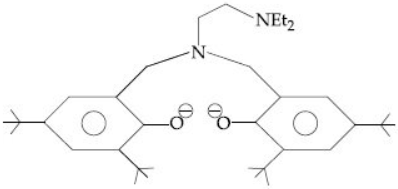## Filters

Sort by :
Clear All
Q

The compound that inhibits the growth of tumors is:

• Option 1)

• Option 2)

• Option 3)

• Option 4)

Application of coordination compounds - Coordination compounds are used for many applications: Extraction process of gold and silver. Used as catalyst in many industrial processes. Purification of metals. Hardness of water.(EDTA) EDTA is used in treatment of lead poisoning. Chelate therapy (removal of toxic proportion of metals in the body) Platinum compounds inhibit the growth...

The degenerate orbitals of   are

• Option 1)

• Option 2)

• Option 3)

• Option 4)

Crystal field splitting in octahedral complex -  orbitals are more energetic as compared to  - wherein     CFSE in octahedral complex -   -      : degenerate orbitals =? Which after CFS bliching energy  divided into 2-set of degenerate orbitals-  & . From option given degenerate orbitals are -  & .  Option 1)             Option 2) Option 3)Option 4)

Complete removal of both the axial ligands (along the z-axis) from an octahedral complex leads to which of the following splitting patterns? ( relative orbital energies not on scale).

• Option 1)• Option 2)• Option 3)• Option 4)Valence bond theory (VBT) - Ligands should have atleast one donor atom with a lone pair of elements. Transition metal atom provide required number of vacant orbitals. These vacant orbitals undergo hybridiation. These hybridised orbitals overlap with filled orbitals of ligands to form coordinaate compound. -     Hybridisation - sp3d2 - square bipyramidal or octahedral  d2sp3 -...

The complex ion that will lose its crystal field stabilization energy upon oxidation of its metal to +3 state is:• Option 1)

• Option 2)

• Option 3)

• Option 4)

Coordination Entity - Comparises of a central metal atom or ion bonded to a  fixed no of ions or molecules - wherein eg:                                            Coordination                      entity             Stability of Complex - Stability of complex is depends on nature of ligands, size of central atom and stability concept. - wherein Stability Stability...

The coordination numbers of Co and Al in  and , respectively, are :

(en = ethane-1, 2-diamine)

• Option 1)

5 and 3

• Option 2)

3 and 3

• Option 3)

6 and 6

• Option 4)

5 and 6

Coordination number - No. of ligands which are bounded directly to metal   - wherein eg:  Coordination No.  eg:   Coordination No.  en is bidentate                     Bidentate ligand  Bidentate ligand  Answer is (5 & 6)   Option 1) 5 and 3 Option 2) 3 and 3 Option 3) 6 and 6 Option 4) 5 and 6

Three complexes,  and

absorb light in the visible region.

The correct order of the wavelength of light absorbed by them is:

• Option 1)

(III) > (I) > (II)

• Option 2)

(III) > (II) > (I)

• Option 3)

(II) > (I) > (III)

• Option 4)

(I) > (II) > (III)

The strength of ligands follows the given order :  is the strongest ligand, thus more energy will be absorbed by it. Thus, lesser wavelength of light it will absorb, because energy is invesely  propotional to wavelength.   will absorb the highest wavelength of light.  correct option is (4) (I) > (II) > (III)Option 1)(III) > (I) > (II)Option 2)(III) > (II) > (I)Option 3)(II) > (I) >...

Consider the hydrated ions of .

The correct order of their spin-only magnetic moments is:

• Option 1)

• Option 2)

• Option 3)

• Option 4)

Spin-only magnetic moment is more for the elements whose number of unpaired electrons are higher.  Spin-only magnetic moment  n (number of unpaired electrons) Now,  =  ( n = 0)  =  ( n = 1)  =  ( n = 2)   =  ( n = 3 )  Correct order is :  So, option (2) is correct.Option 1)Option 2)Option 3)Option 4)

The species that can have a trans-isomer is:

( en = ethane-1,2-diamine, ox = oxalate)

• Option 1)

• Option 2)

• Option 3)

• Option 4)

(1)  :  :  hybridised , therefore no trans-isomer is possible. (2)  : Bidentate ligand will be co-ordinating at  in square planar complex.                                  Thus, no trans-isomer is possible. (3)  : In this compound as well, no trans-isommer is possible. (4)  In this compound both trans and cis are possible.  option (4) is correct.Option 1)Option 2)Option 3)Option 4)

The crystal field stabilization energy (CFSE) of   and  , respectively , are :

• Option 1)

• Option 2)

• Option 3)

• Option 4)

(a) CFSE of   is weak ligands so pairing not take place       eg. CFSE=  (b) CFSE of                                    weak field ligand pairing not take place & also have tetrahedra geometry.               e.g.    ,          CFSE=Option 1)Option 2)        Option 3)Option 4)

The maximum possible denticities of a ligand given below towards a common transition and inner - transition metal ion , respectively, are  :• Option 1)

8 and 6

• Option 2)

6 and 8

• Option 3)

6 and 6

• Option 4)

8 and 8

Coordination number - No. of ligands which are bounded directly to metal   - wherein eg:  Coordination No.  eg:   Coordination No.  en is bidentate         Co-ordination number towards a common transition element (3d) =  6 CN of inner transition element = 8 to 12 so option 6,8 will be correct. Option 1) 8 and 6 Option 2) 6 and 8 Option 3) 6 and 6 Option 4) 8 and 8

The correct order of the spin-only magnetic moment of metal ions in the following law-spin complexes,

is:

• Option 1)

• Option 2)

• Option 3)

• Option 4)

Configuration                No.of unpaired electron                    Option 1)Option 2)Option 3)  Option 4)

The following ligand is :• Option 1)

• Option 2)

bidentate

• Option 3)

tridentate

• Option 4)

the oxidation number of Mn in the product of the alkaline oxidative fusion of MnO2 is x. then the value of x is?
Let the oxidation state of Mn in MnO42– is x. So, x + 4 (–2) = –2 or x=6

Match the metal (column I) with the coordination compound (s) / enzyme (s)(column II):

(column I)                                (column II)

Metal                                         Coordination compound (s)/enzyme(s)

(A) Co                                    (i) Wilkinson catalyst

(B) Zn                                     (ii)Chlorophyll

(C) Rh                                    (iii)Vitamin

(D) Mg                                    (iv) Carbonic anhydrase

• Option 1)

( A) - (ii) ; (B) - (i) ; (C) - (iv) ; (D) - (iii)

• Option 2)

( A) - (iv) ; (B) - (iii) ; (C) - (i) ; (D) - (ii)

• Option 3)

( A) - (iii) ; (B) - (iv) ; (C) - (i) ; (D) - (ii)

• Option 4)

( A) - (i) ; (B) - (ii) ; (C) - (iii) ; (D) - (iv)

Organo Metalics - Compound in which metal carbon bond is present is known as organo metalic compound. - wherein As we know that vitamin B12 (cynobalamin) Carbonic anhydrase wiliknson catalyst Chlorophyll    Option 1)( A) - (ii) ; (B) - (i) ; (C) - (iv) ; (D) - (iii)Option 2)( A) - (iv) ; (B) - (iii) ; (C) - (i) ; (D) - (ii)Option 3)( A) - (iii) ; (B) - (iv) ; (C) - (i) ; (D) - (ii)Option...

The coordination number of Th in  is :

• Option 1)

14

• Option 2)

8

• Option 3)

10

• Option 4)

6

Coordination number - No. of ligands which are bounded directly to metal   - wherein eg:  Coordination No.  eg:   Coordination No.  en is bidentate  Option 1)14Option 2)8Option 3)10Option 4)6

The number of bridging CO ligands (s) and Co-Co bond (s) in Co2 (CO)8, respectively are :

• Option 1)

2 and 1

• Option 2)

4 and 0

• Option 3)

0 and 2

• Option 4)

2 and 0

Oxidation number - It is represented by roman numeral in parenthesis following the name of coordination entity. - wherein Eg.  x-4 = -2 x= 2 Structure  No.of CO-CO bond =1  No. of Bridging carbon =2        Crystal Field Theory - According to this theory, metal and ligand are considered as point charges. This theory deals with electrostatic force of attraction means the talks about the ionic...

A reaction of cobalt (III) chloride and ethylenediamine in a 1:2 mole ratio generates two isomeric poducts A (voilet coloured) and B (green coloured) A can show optical activity but B optically inactive. what type of isomers does A and B represent?

• Option 1)

Ionisation isomers

• Option 2)

Coordination isomers

• Option 3)

Geometrical isomers

• Option 4)

Geometrical Isomers - This is due to the difference in relative position of the ligands attached to the central metal atom with respect to each other -   As we have learned in isomerism when one is optically active and one is optically inactive A and B are geometrical isomers .Option 1)Ionisation isomersOption 2)Coordination isomersOption 3)Geometrical isomersOption 4)Linkage isomers

The difference in the number of unpaired electrons of  a metal ion in its high-spin and low-spin octahedral complexes is two ,The mwtal ion is:

• Option 1)

• Option 2)

• Option 3)

• Option 4)

Strong Field Ligand - These are the ligands which pair the electron in inner d-orbital and makes required d-orbitals empty/available.  - wherein For example: CO, CN etc     Weak field Ligand - These are the ligands which do not pair the electron in inner d-orbital. - wherein For example:  etc        Option 1)      Option 2)Option 3)Option 4)

The magnetic moment of an octahedral homoleptic Mn (II) complex is 5.9 BM. The suitable ligand for this complex is :

• Option 1)

CO

• Option 2)

ethylenediamine

• Option 3)

NCS-

• Option 4)

CN-

CFSE in an octahedral complex -   -   Magnetic Moments (spin only) -  where n= number of unpaired electron. - wherein Number of unpaired  and corresponding magnetic moments As we have learned in magnetic moment   therefore no. of unpaired cation   configuration only possible for relatively weak ligand . therefore       Option 1)COOption 2)ethylenediamine Option 3)NCS-Option 4)CN-

The total number of isomers for a square planar complex   is :

• Option 1)

16

• Option 2)

8

• Option 3)

4

• Option 4)

12

Isomers - Isomers are two or more compounds that have the same chemical formula but a different arrangement of atoms -       As we have learnt in square planar complx. The total number of isomers for the complex is 12.   Option 1)16Option 2)8Option 3)4Option 4)12
Exams
Articles
Questions